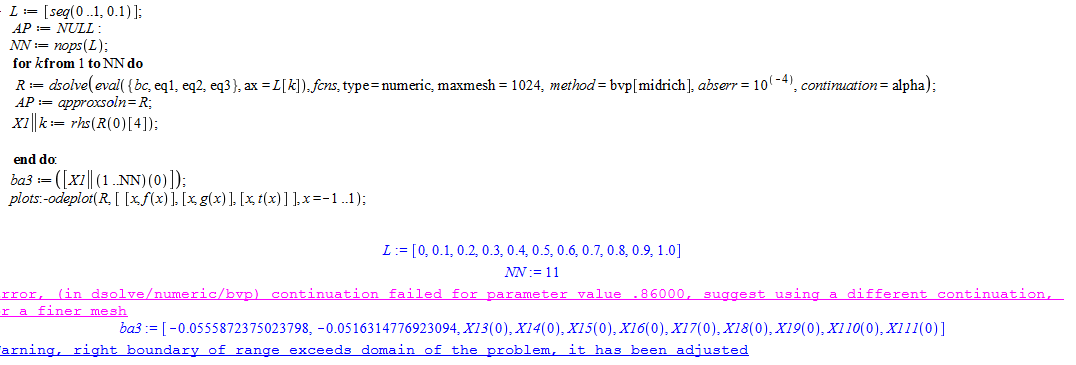<

javid basha jv

## 120 Reputation

4 years, 355 days

## Contour labels in the graph...

Is this possible to add contour labels to the graph?

Tim Leslie 7597  suggested the above-mentioned link,  there it is explained for algebraic functions only.

In this case, we have the data in the matrix form.

Kindly help me to shoot out this problem.

## How to add the labels in the graph...

Dear @tomleslie

Is this possible to add the contour sequence and how to incorporate the labels in the graph?

## How to get the solution...

Dear @tomleslie

Is there any possibility to get a solution to this problem?

## Please find this attachment....

Dear @tomleslie

Please find this attachment.
This paper problem only I am trying to get a solution.

Paper: perturbation.pdf

In this paper, equations 14-16 are solved using the perturbation function 20a-20e with the help of 17a, 17b and 18.

After applying the boundary condition and perturbation function in equations 14-16, they displayed the final form in equations 21-23.

## Results are not matching....

I have tried the below-mentioned problem. But the outcomes are not matching. I don't know where the mistakes happen.

Equation:Condition:perturbation expansion:Outcome:Result:## Solution for this PDE Via Perturbation...

Is there any possibility to get a solution to this problem?

## Nice outcome....

Dear @tomleslie

The outcome looking good.
Thanks for your wonderful support.

## Thanking you for your attention...

Dear @tomleslie

Thanks for your notable support.

The outcome looking nice.

How to find values for R(0..1)

Only one point the value is coming.

X1[L[k]]:=rhs(R[L[k]](0..1));

## Thanks for the response....

Dear @tomleslie

I have applied sequences for "ax "
But solution is not coming.

How to get a result for the sequence of values.Dear maple users,
I hope anyone of the users answers this question.

## Thanks for the response....

Dear @Kitonum

And many thanks for your support.

## how to change axis label....

Dear @Kitonum

Greetings.

How to change x and y coordinate labels.
η and r instead of x and y.

A3 := .25*y*(-6*x^2+6*x-2.477250468*x*(x-1)^2-2.477250468*x^2*(x-1)-1.476663599*x*(x-1)^3-2.214995399*x^2*(x-1)^2+.3837076420*x*(x-1)^4+.7674152840*x^2*(x-1)^3+1.049305257*x*(x-1)^5+2.623263142*x^2*(x-1)^4+1.470504325*x*(x-1)^6+4.411512974*x^2*(x-1)^5+2.062933702*x*(x-1)^7+7.220267957*x^2*(x-1)^6+1.610136961*x*(x-1)^8+6.440547843*x^2*(x-1)^7+.6577852166*x*(x-1)^9+2.960033475*x^2*(x-1)^8);

ContoursWithLabels(A3, 0 .. 1, 0 .. 10, {seq(0.1..5,0.2)}, [color = black,thickness = 2, axes = box, size=[450,450]], Coloring = [colorstyle = HUE, style = surface],[labels = ["eta", "r"],labeldirections = [horizontal, vertical], labelfont = ['TIMES', 'BOLDOBLIQUE', 16]]);